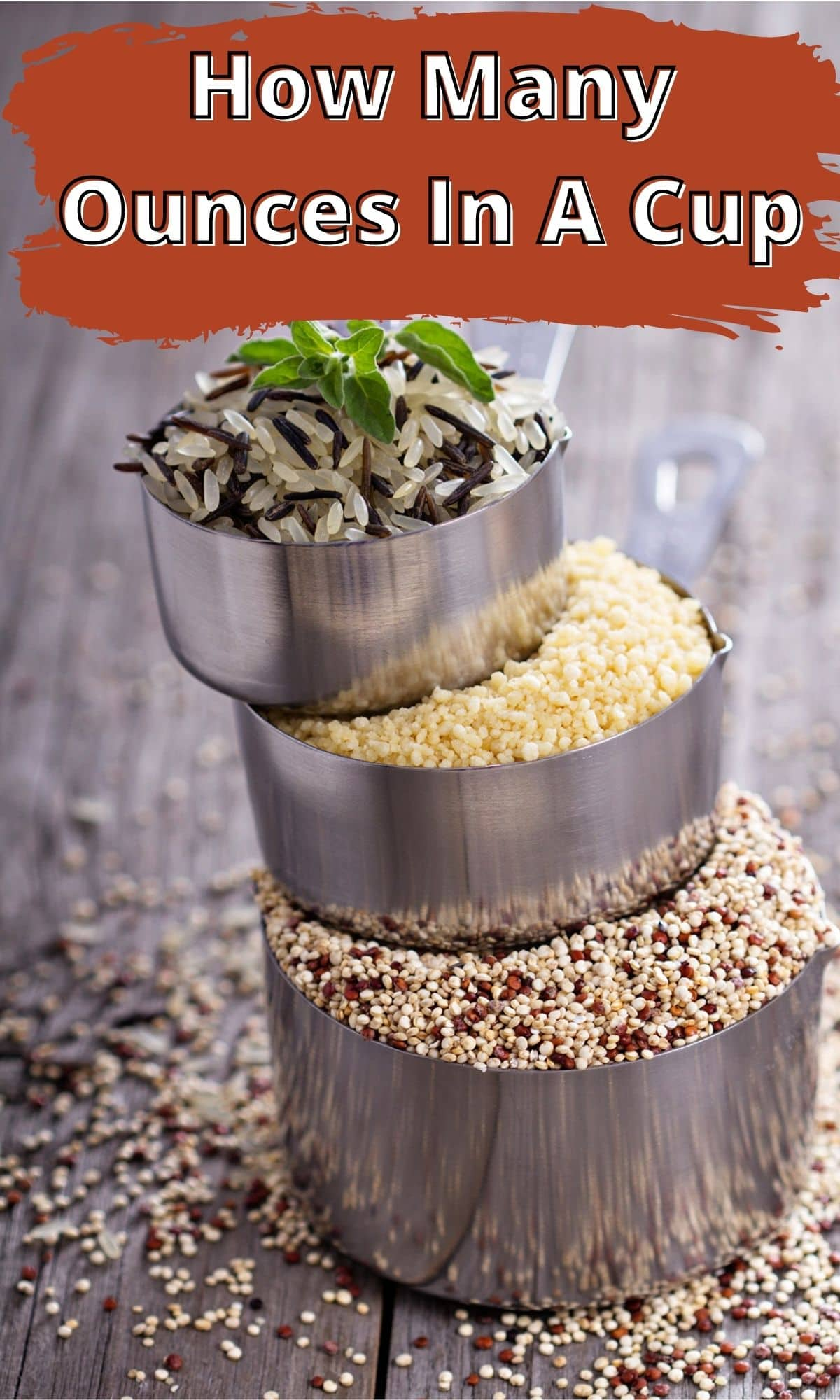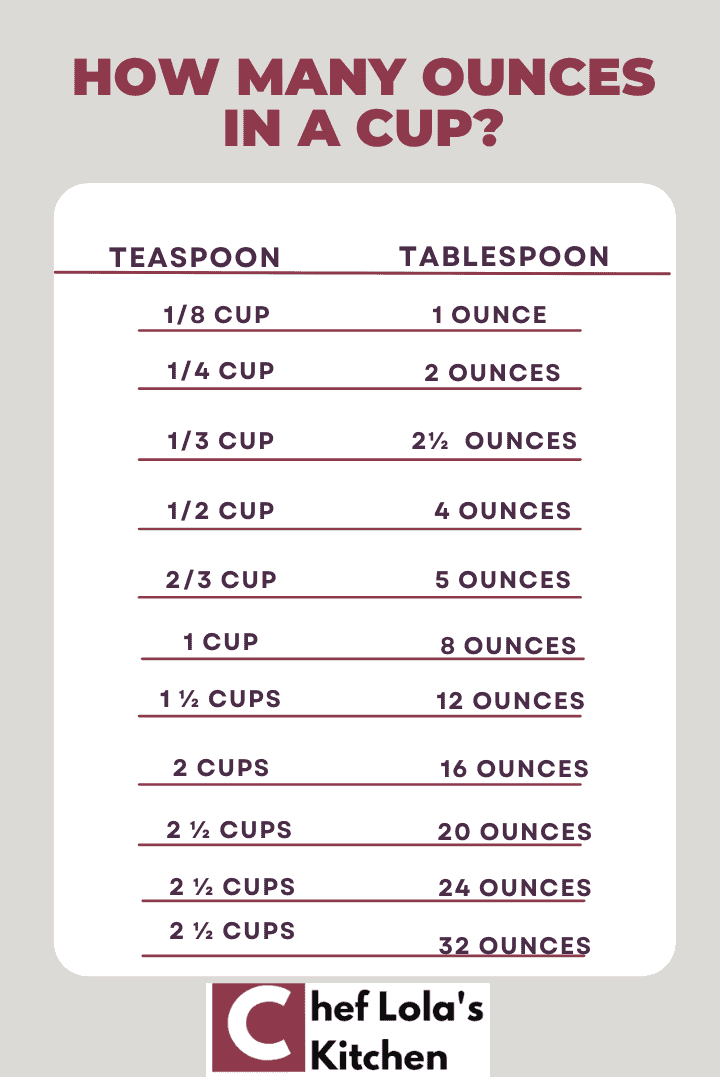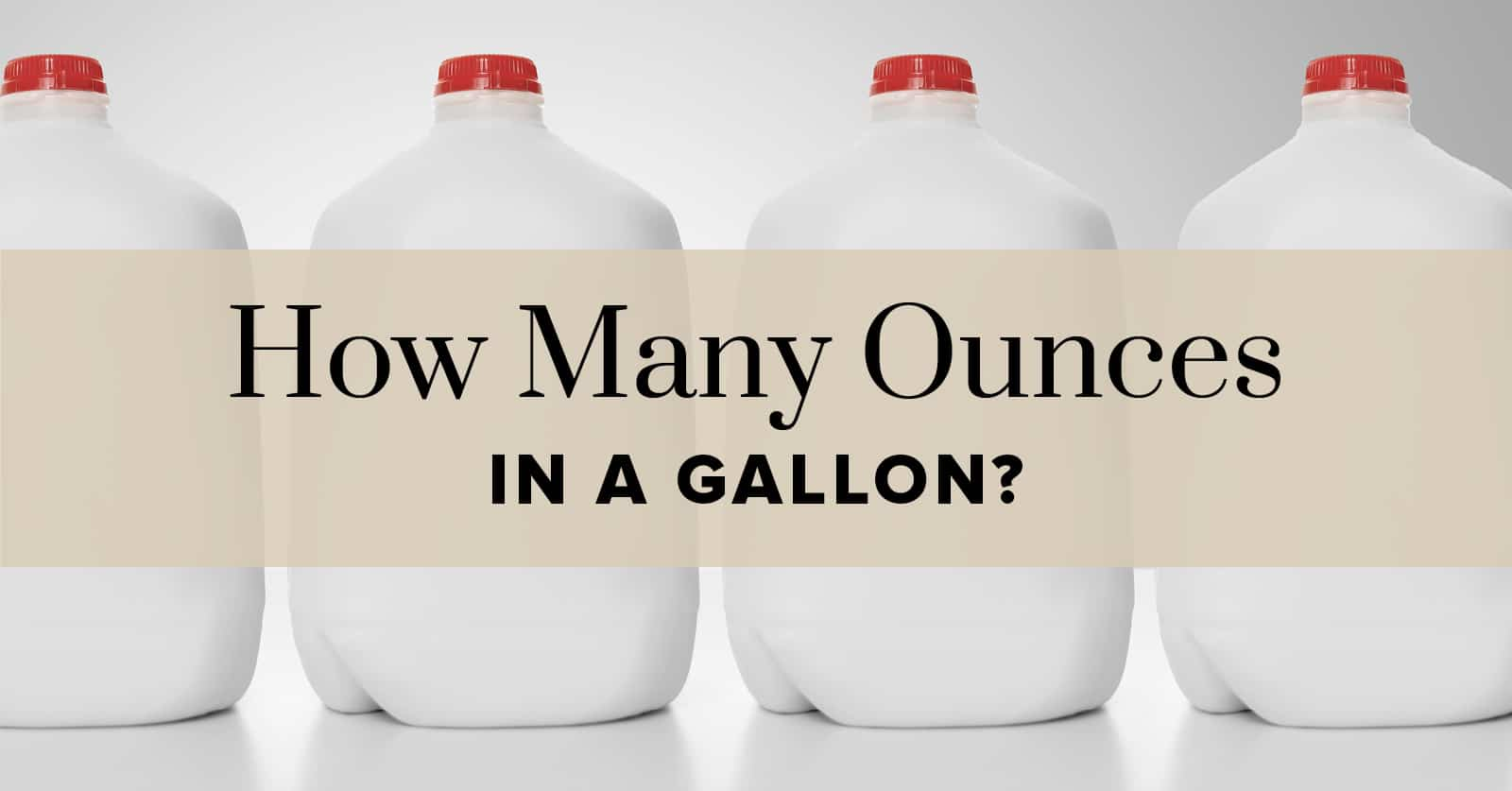# How many oz in a cup ?

An ounce is a unit of mass, weight, or volume that is derived almost exactly from the uncia, an Ancient Roman unit of measurement. So, the question is for us to figure is how many oz in a cup?

The avoirdupois pound is 6 times an avoirdupois ounce (exactly 28.349523125 g); this is the US customary and British imperial ounce. In the United States, it is primarily used to measure packaged foods and food portions. It also measures postal items, the area density of fabric and paper, boxing gloves, and so on.

Historically, various ounces measuring mass or volume were in use in various jurisdictions by various trades and at various times in history.

Moreover, there are two types of ounces that we measure: dry and fluid. Because dry and liquid ounces are not the same, you must measure dry ingredients by weight. We use fluid ounces to measure liquid ingredients. Because there is a significant difference between fluid and dry ounces, always double-check the ingredients and accurately measure before beginning any recipe.

The cup is a cooking volume measurement that has common usage in cooking and serving sizes. In the United States, it is traditionally equal to one-half of a US pint (236.6 ml). Standard measuring cups, with a metric cup being 250 ml, may be used because actual drinking cups may differ significantly from the size of this unit.

A cup as a unit of measurement varies from country to country. In addition to the US and metric cups, there are Russian, Canadian, Japanese, Latin American, and even coffee cups. A Legal cup is even a unit of measurement. The Legal cup is 240 mL and is used for nutrition labelling in the United States.

1 cup equals 8 fluid ounces. In terms of dry ounces, one cup equals 4.5 dry ounces.

## How many dry oz in a cupWhat are fluid ounces in terms of cups? 1 cup contains exactly 8 fluid ounces (fl oz). Keep in mind that 1 ounce equals 0.125 cups. For this conversion, simply multiply the number of ounces by 0.125 cups.

Converting dry ounces to cups isn’t as simple as one might think. Some take up more space than others, depending on factors such as shape, size, and form. A cup is approximately 4.5 ounces when properly measured. However, this varies from dry substance to dry substance. When it comes to measuring dry goods in terms of cups, one must take a variety of factors into consideration.

## How many grams in a cup

A gram (originally gramme) is a unit of mass equal to one-thousandth of a kilogramme.

Today, the gramme is the most common unit of measurement for non-liquid ingredients in cooking and grocery shopping around the world. Volume rather than mass can be used to measure liquid ingredients.

Many standards and legal requirements for nutrition labels on food products require relative contents to be stated per 100 g of product, with the resulting figure also being readable as a percentage.

Read Also: How many quarts in a gallon ?

The cup is a cooking volume measurement that is frequently used in cooking and serving sizes. It is traditionally equal to one-half of a US pint in the United States (236.6 ml). Because actual drinking cups may differ significantly from the size of this unit, standard measuring cups, with a metric cup being 250 ml, may be used.

A cup can have several gram equivalents in terms of mass. Moreover, it totally depends on the kind of element you are measuring, its density, its nature, and more. Moreover, since there are several variations of just the cup measure, it also depends on the cup unit you mean.

## How many ounces in a cup and halfFirst of all, remember that there are two types of ounces: fluid and dry. They are not equal in measurement. So if you want to convert ounces to some other unit, make sure you know the kind of ounce you are converting.

1 cup equals 8 fluid ounces or 8 fl oz. In terms of dry ounces, it equals 4.5 dry ounces or dry oz. Therefore, if we apply simple arithmetic, we will find half a cup and a half to be equal to 4 fluid ounces and 2.25 dry ounces.

A cup and a half is simply the sum of 1 cup and half a cup. So, ounces in terms of cups would be the same too. A cup and a half equals 8 plus 4, that is, 12 fluid ounces. Again, in terms of dry ounces, a cup and a half are 6.75 dry ounces.

## How many oz in a gallonIn the Imperial (UK) and customary units systems, a gallon is a volume unit. The gallon currently has one imperial definition and two US customary definitions (liquid and dry). Throughout history, there have been numerous definitions and redefinitions.

A quart is a volume unit in English equal to one-quarter gallon. They are all roughly equivalent to one litre. The imperial gallon is approximately 20% larger than the American gallon. This means they are not the same and can be used to refer to the same thing.

One Imperial gallon contains 160 fluid ounces. One US gallon contains 128 fluid ounces.

## How many tablespoons in a cup

A tablespoon is a large spoon (tbsp., Tbsp., Tb., or T.). In many English-speaking regions, the term now refers to a large serving spoon, but in others, it refers to the largest type of eating spoon.

By extension, the term is also common in cooking as a volume measurement. To distinguish it from the utensil, they usually abbreviate it as tbsp. or Tbsp. Moreover, they also sometimes refer to it as a tablespoonful. A tablespoon in the United States is approximately 14.8 ml, a tablespoon in the United Kingdom and Canada is precisely 15 ml, and an Australian tablespoon is 20 ml.

The capacity of the utensil (rather than the measurement) is determined by preference rather than law or custom. It may or may not be significantly less than the measurement.

Tablespoons and cups as measurements have a very close link. One US cup contains 16 US customary tablespoons, which is the relationship between the most common tablespoon and cup measure, the US customary tablespoon and the US customary cup.

## How many oz in a cup of butter

One cup equals 4.5 dry ounces. If you measure one cup of butter in terms of ounces, it amounts to roughly 4.5 ounces.

## How many cups in a pintIn both the Imperial and US customary measurement systems, the pint is a volume or capacity unit. In both of those systems, it is traditionally one-eighth gallon. The British Imperial pint is approximately 20% larger than the American pint because the two systems are defined differently. Almost every other country has adopted the metric system, so while some continue to use traditional units called pint (for example, for beverages), the volume varies according to regional custom.

In terms of cups, one pint equals two cups. The formula is very simple. If you want to convert a certain number of pints to cups, just multiply the number of pints you want to convert to cups by 2. For example, if you want to know the value of 7 pints in terms of cups, multiply 7 by 2. 2 times 7 equals 14, so 7 pints equals 14 cups.

## How many oz in a cup of waterSince water is fluid, the ounces-to-cups conversion for water would be fluid ounces-to-cups conversion. Thus, since one cup equals 8 fluid ounces equals 1 cup, 1 cup would contain 8 ounces of water.

## How many ml in a cupThe litre (international spelling) or litre (American English spelling) is a volumetric unit for metric measurements.

Litres are the most common units for items that one measures by the capacity or size of their container (such as fluids and solids that can be poured), whereas cubic metres (and derived units) are the most common units for items one can measure by their dimensions or displacements. The litre is also frequently used in some calculated measurements, such as density (kg/L), allowing for easy comparison with water density.

One litre equals 1000 millilitres or 1000 ml. In other words, one ml is one-thousandth of a litre.

One US cup is equal to 236.588 ml. In approximate terms, it is equal to 236.6 ml.

## How many oz in a cup of coffeeCoffee is a beverage whose primary ingredient is roasted coffee beans. Coffee, which is dark in colour, bitter, and slightly acidic, has a stimulating effect on humans, owing to its caffeine content. It is the world’s most popular hot beverage.

The standard conversion is eight ounces per cup, but a cup of coffee is only four ounces. To make matters worse, brewing coffee requires slightly more water by volume than the amount of coffee produced, implying that brewing a 4-ounce cup of coffee requires approximately 5 ounces of water. Coffee grounds absorb some water during the brewing process, resulting in less coffee by volume than the amount of water used.

On the carafe of many automatic drip coffee makers, there are two sets of lines, one for measuring water and the other for measuring brewed coffee. Consider a 12-cup coffee maker as an example. Typically, the carafe will have markings at 4, 6, 8, 10, and 12 that correspond to 20, 30, 40, 50, and 60 ounces of water. Remember that 20 ounces of water will yield approximately 16 ounces of brewed coffee.

Let’s go over a concrete example to make sure everything is clear. Assume you want to make two 8-ounce mugs of coffee. To brew 16 ounces of coffee, use 20 ounces of water, which means filling your carafe to the 4 line.

Therefore, for coffee, the conversion from ounces to cups is not simple. It ultimately falls down the recipe and your tastes and preferences.

## How many oz in a cup FAQs

### Is 1 cup the same as 8 oz?

Yes. 1 cup does equal 8 ounces, but the fluid ounces, not just any ounce. This distinction is important because the other type of ounce, dry ounce, has a different measurement. 4.5 dry ounces equal one cup.

### How many dry ounces in a cup?

One cup contains 4.5 dry ounces. The other ounce, the fluid ounce, has a different measure. In terms of fluid ounces, one cup is equal to 8 fluid ounces.

### Is a cup 16 oz or 8 oz?

One cup is 8 fluid ounces or 4.5 dry ounces. By simple arithmetic, we know that 2 cups are equal to 16 fluid ounces or 4.5 dry ounces.

### Is 4 oz half a cup?

One cup is 8 fluid ounces or fl oz. So, by basic mathematics, half a cup is 4 fluid ounces.

### Is dry measure cup the same as liquid?

Technically speaking, they are the same. They both have the same volume measurement. There is no conversion from liquid to dry measuring cups. Cups in dry measuring cups equal cups in liquid measuring cups.

### What is the difference between dry ounces and wet ounces?

Dry ounces and fluid or wet ounces are not the same. As their names suggest, both measure different types of substances. Dry ounces are to measure dry, solid things. On the other hand, fluid ounces measure liquids. In terms of measurement, they are different too. If we see their values in terms of cups, one cup is 8 fluid ounces and, in terms of dry ounces, 4.5 dry ounces.

### Which ingredient is measured incorrectly most often?

The most commonly incorrectly measured ingredient is flour. Therefore, try to use the “spoon & level” method when you are measuring bread flour, cake flour, all-purpose flour, or any other flour on the market.

### What are the three measurement errors?

The three types of measurement errors are gross error, systematic error, and random error.